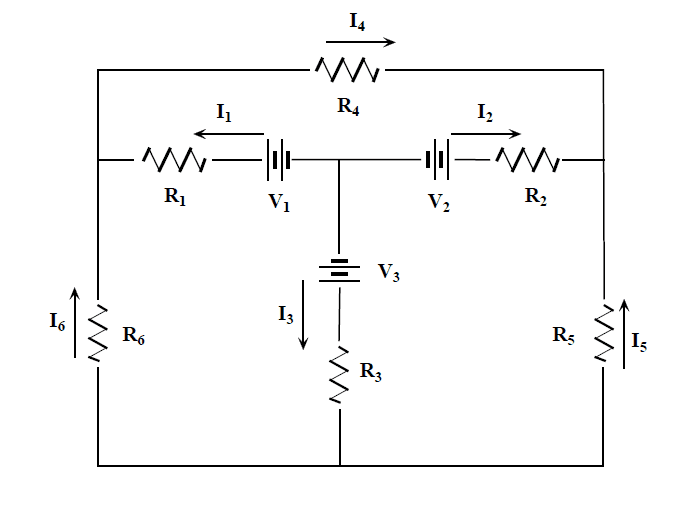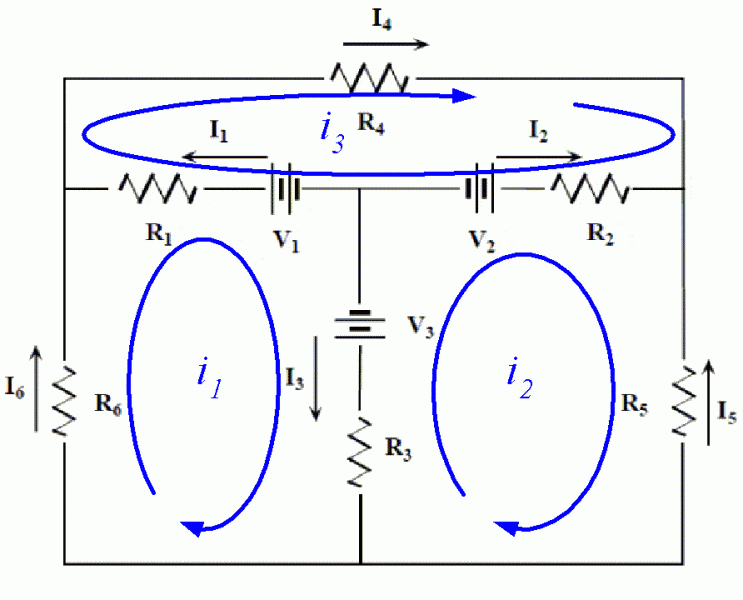# Multiple Power Source Circuit Analysis

## Homework Statement

Compute all the currents labeled using the mesh loop method.## Homework Equations

Parallel Resistors
1/Rt=1/R1+1/R2...+1/Ri
Series Resistors
R1+R2...+Ri

## The Attempt at a Solution

My attempt at the kvl and kcl analysis, I think I did this wrong because I can't solve for the variables[/B]
v1 - (I1 - I6 - I3)*r1 - (-I6 - I3 + I1)*r6 - (-I3 - I6 + I1)*r3 - v3=0
v2 - (I2 - I5 - I3)*r2 - (-I5 - I3 + I2)*r5 - (-I3 - I5 + I2)*r3 - v3=0
v1 - (I1 + I4 - I2)*r1 - (I4 - I2 + I1)*r4 - (-I2 + I1 + I4)*r2 - v2=0
I4 = I1 - I2
I6 = I3 - I1
I5 = I3 - I1

This is my first time doing this so I'm sure I did this wrong. Any help is appreciated!

phinds
Gold Member
2021 Award
Your equations are WAY too complex, bringing in terms that don't belong.

Just take the first one, for example, and your inclusion of I1 and I6 as multipliers for R1 when only I3 is flowing through R1

They are all like that.

Ok, then I'm confused.

So for the left area it goes:
V3-I3*R3-I3*R6-I3*R1-V1=0

So then what happens to I1 and I6?

phinds
Gold Member
2021 Award
There is a current designator that shows the current through R3. It is I3. There IS no other current going through that branch (R3)

There is a current designator that shows the current through R6. It is I6. There IS no other current going through that branch (R6)

You are making things more complicated than they need to be.

Ok. Trying to simplify this and knowing that the current through each resistors is ONLY it's corresponding current number.

V3-I3*R3-I6*R6+I1*R1-V1=0
V3-I3*R3-I5*R5+I2*R2-V2=0
V1-I1*R1-I4*R4+I2*R2-V2=0

Am I at least on the right track?

phinds
Gold Member
2021 Award
I haven't checked in detail but yes, that's exactly the kind of thing you want to see

phinds
Gold Member
2021 Award
Glad to help. I love these easy onesgneill
Mentor
Mesh loop analysis is based upon mesh currents. Each loop is assigned a "brand new" current of its own which circulates within that loop alone. KVL equations are written for each loop using these new currents and solved.Thus in the above we define mesh currents ##i_1##, ##i_2##, and ##i_3##.

Note that more than one mesh current can flow in a given component (the ones that border two loops). In the above circuit R1 borders loops 1 and 3, so that it conducts ##i_1## and ##i_3## (flowing in opposite directions).

After solving for the mesh currents you can then go back and form any desired current in the circuit by noting which mesh currents contribute to them. For example, in the above circuit ##I_3 = i_1 - i_2##, and ##I_5 = -i_2##.

There's a very good reason for defining and solving for mesh currents in this way. One can write the loop equations in matrix form easily by inspection -- there's a very simple procedure. It's a virtually foolproof way to obtain the circuit equations quickly and without error.

phinds
OOPS ... gneill obviously has it right. I was so focused on one thing that I lost track of another.